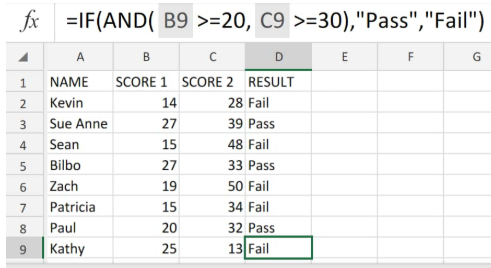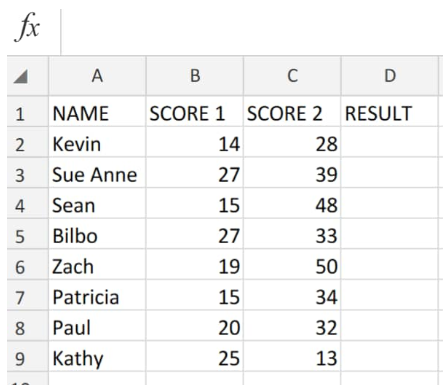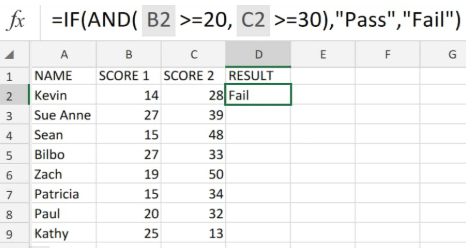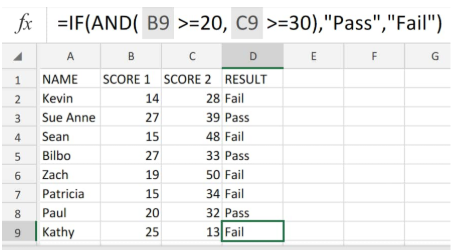Get instant live expert help with Excel or Google Sheets“My Excelchat expert helped me in less than 20 minutes, saving me what would have been 5 hours of work!”

#### Post your problem and you’ll get expert help in seconds.

Your message must be at least 40 characters
Our professional experts are available now. Your privacy is guaranteed.

# How to use Conditional Formulas in Excel – Excelchat

Excel allows us to utilize its IF, AND, OR, and NOT functions to generate conditional formulas which test for true or false conditions and also make logical comparison between different expressions.

These Excel conditional formulas essentially allow us to create basic logical If (this), then (that)arguments.Figure 1. of Excel Conditional Formulas

## Formula Syntax

`=IF (logical_test, [value_if_true], [value_if_false])`

• logical_test = A logical expression or value which is to be tested for being TRUE or FALSE.
• value_if_true (optional) = The specified value to return if the logical_test is TRUE.
• value_if_false – (optional) = The specified value to return if the logical_test is FALSE.

## How to Write a Conditional Formula in Excel

Because making logical comparisons among varying expressions and testing for true or false conditions are common to lots of tasks, we can make use of the IF and AND functions to create our own conditional formulas excel.

1. We begin by entering the data available to us under well-labelled columns of our worksheet;Figure 2. of Excel Conditional Formulas

In the worksheet above, our purpose is to ascertain if each student that took two separate exams, met the criteria specified by us; which in this case is a certain score set for each test.

2. The conditional formatting if formula in cell D2 of our worksheet is as follows;
`=IF(AND(B2>=20,C2.>=30),”Pass”,”Fail”)`

meaning , if a student obtained a specific score in both tests (20 and 30), they would either pass or fail.Figure 3. of Excel Conditional Formulas

3. Modify and copy the conditional formatting if formula in cell D2 down into the other cells in column D for the other conditional results:Figure 4. of Excel Conditional Formulas